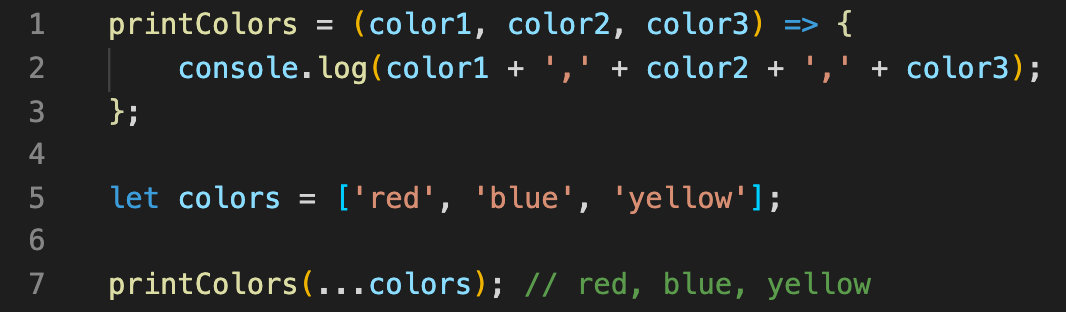1629649881

# Hướng dẫn học JavaScript ES6 - Spread Operator

Hướng dẫn học JavaScript ES6 - Spread Operator

#javascript #es6

## Buddha Community1622036598

## JavaScript compound assignment operators

JavaScript is unarguablly one of the most common things you’ll learn when you start programming for the web. Here’s a small post on JavaScript compound assignment operators and how we use them.

The compound assignment operators consist of a binary operator and the simple assignment operator.

The binary operators, work with two operands. For example a+b where + is the operator and the a, b are operands. Simple assignment operator is used to assign values to a variable(s).

It’s quite common to modify values stored in variables. To make this process a little quicker, we use compound assignment operators.

They are:

• +=
• -+
• *=
• /=

You can also check my video tutorial compound assignment operators.

Let’s consider an example. Suppose price = 5 and we want to add ten more to it.

var price = 5;
price = price + 10;

We added ten to price. Look at the repetitive price variable. We could easily use a compound += to reduce this. We do this instead.

price += 5;

Awesome. Isn’t it? What’s the value of price now? Practice and comment below. If you don’t know how to practice check these lessons.

Lets bring down the price by 5 again and display it.
We use console.log command to display what is stored in the variable. It is very help for debugging.
Debugging let’s you find errors or bugs in your code. More on this later.

price -= 5;
console.log(price);

Lets multiply price and show it.

price *=5;
console.log(price);

and finally we will divide it.

price /=5;
console.log(price);

If you have any doubts, comment below.

#javascript #javascript compound assignment operators #javascript binary operators #javascript simple assignment operator #doers javascript1594059180

## The Rest Parameter

A Rest Parameter is used as the last argument of a function declaration. It is enabling the user to specify any number of parameters.

``````function sumUp(...nums) {
return nums.reduce((acc, cur) => acc = Number(acc + cur), 0);
}

console.log(sumUp(1, 2, 3));
// -> 6
``````

A function can also have common parameters as long as the Rest Parameter is the last one.

``````	function myHero(name, level, ...abilities) {
return `My hero \${name} on level \${level} has the following abilities: \${abilities.join(", ") || 'none'}.`;
}

console.log(myHero('Arthas', 55, 'Attack', 'Blizzard'));
// -> My hero Arthas on level 55 has the following abilities: Attack,Blizzard.

console.log(myHero('Thrall', 1));
// -> My hero Thrall on level 1 has the following abilities: none.
``````

## Rest Parameter vs. Arguments

Arguments is an Array-like Object; we cannot directly apply Array-functions to it. We have to make use of the Spread Operator to unfold the Arguments Object in an Array first. For instance: In the last example, we could apply the join method directly to our `abilities` parameter.

``````function sumUp() {
return [...arguments].reduce((acc, cur) => acc = Number(acc + cur), 0);
}

console.log(sumUp(1, 2, 3));
// -> 6
``````

#spread-operator #es6 #rest-parameter #learn-javascript #javascript-basics #javascript1629649881

## Hướng dẫn học JavaScript ES6 - Spread Operator

Hướng dẫn học JavaScript ES6 - Spread Operator

#javascript #es61593530460

## JavaScript: Spread Operator and Rest Parameter (ES6)

The spread operator and rest parameter are two other powerful features introduced in ECMAScript 2015 and they allow us to write more concise and flexible code like most of the other ES6 features do. The several different ways you can use spread and rest syntax will be covered in this article.

The spread operator allows us to expand the collected elements of an iterable data type like strings, arrays, or objects into individual elements in places. It helps to create a list of elements from a list by spreading out the values of an iterable where zero or more arguments (function calls) or elements (array literals) or key-value pairs (object literals) are expected.

1. In Function Calls:

The spread operator can be used to take elements of an array and pass them as a list of arguments into a function. Let’s take a look at how it works:The elements of an array are passed to a function as arguments

The function `printColors` takes in three parameters and we want to pass three arguments stored in `colors` array. When we call the function, the spread operator unpacks the passed array of arguments, `‘red’``‘blue’`, and `‘yellow’`.